# Test: Electronic Devices And Circuits - 4

## 25 Questions MCQ Test Electronic Devices | Test: Electronic Devices And Circuits - 4

Description
This mock test of Test: Electronic Devices And Circuits - 4 for Electrical Engineering (EE) helps you for every Electrical Engineering (EE) entrance exam. This contains 25 Multiple Choice Questions for Electrical Engineering (EE) Test: Electronic Devices And Circuits - 4 (mcq) to study with solutions a complete question bank. The solved questions answers in this Test: Electronic Devices And Circuits - 4 quiz give you a good mix of easy questions and tough questions. Electrical Engineering (EE) students definitely take this Test: Electronic Devices And Circuits - 4 exercise for a better result in the exam. You can find other Test: Electronic Devices And Circuits - 4 extra questions, long questions & short questions for Electrical Engineering (EE) on EduRev as well by searching above.
QUESTION: 1

### An increase in junction temperature of a semiconductor diode

Solution:

Reverse saturation current doubles for every 10ºC rise in junction temperature.

QUESTION: 2

Solution:
QUESTION: 3

### Generally, the gain of a transistor amplifier falls at high frequency due to the

Solution:
QUESTION: 4

Which of these has a layer of intrinsic semiconductor?

Solution:

The intrinsic layer is in between p and n layers.

QUESTION: 5

Assertion (A): When Diode used as rectifier the reverse breakdown voltage should not be exceeded.

Reason (R): A high inverse voltage can destroy a p-n junction.

Solution:

PIV is an important parameter in rectifier operation.

QUESTION: 6

A Si sample is doped with a fixed number of group N impurities. The electron density n is measured from 4 K to 1200 k for the sample. Which one of the following is correct?

Solution:
QUESTION: 7

Assertion (A): In design of circuit using BJT, a derating factor is used.

Reason (R): As the ambient temperature increases, heat dissipation becomes slower.

Solution:

Increase of ambient temperature lowers the heat dissipation capacity.

Therefore derated operation of BJT is necessary lest BJT should be destroyed.

QUESTION: 8

If the drift velocity of holes under a field gradient of 100 V/m is 5m/sec. Their mobility is

Solution:

E = μ . Vd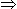μ =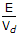.

QUESTION: 9

In a P type silicon sample, the hole concentration is 2.25 x 1015 / cm3. If the intrinsic carrier concentration is 1.5 x 1010/ cm3 the electron concentration is

Solution:

Electron concentration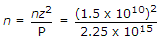.

QUESTION: 10

The behaviour of a JFET is similar to that of

Solution:

Both JFET and vacuum triode are voltage controlled devices.

QUESTION: 11

What is the effect of cut in voltage on the wave form of output as compared to input in a semiconductor diode?

Solution:

Since the duration of output waveform is less than 180º, output voltage is less than input voltage.

QUESTION: 12

As temperature increases the number of free electrons and holes in an intrinsic semiconductor

Solution:

Increase of temperature increases conductivity of semiconductors.

QUESTION: 13

At room temperature kT = 0.03 eV.

Solution:
QUESTION: 14

Assertion (A): A JFET behaves as a resistor when VGS < VP.

Reason (R): When VGS < VP, the drain current in a JFET is almost constant.

Solution:
QUESTION: 15

In a reverse biased P-N junction, the current through the junction increases abruptly at

Solution:
QUESTION: 16

Dielectric strength of polythene is around

Solution:
QUESTION: 17

Resistivity of hard drawn copper is

Solution:
QUESTION: 18

The channel of JFET consists of

Solution:

The channel in JFET may be n type or p type. If channel is n type, gate is p type and if channel is p type gate is n type.

QUESTION: 19

In a bipolar junction transistor adc = 0.98, ICO= 2 μA and 1B = 15 μA. The collector current IC is

Solution: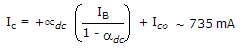QUESTION: 20

The voltage across the secondary of the transformer in a half wave rectifier with a shunt capacitor filter is 50 volts. The maximum voltage that will occur on the reverse biased diode will be

Solution:
QUESTION: 21

Assertion (A): The forward resistance of a p-n diode is not constant.

Reason (R): The v-i characteristics of p-n diode is non-linear.

Solution:

If v-i characteristics is non linear, the ratio v-i is not constant.

QUESTION: 22

For a photoengraving the mask used is

Solution:

The mask that is prepared first is called the master mask made from glass substrates covered by thin chromium film.

From the master masks are prepared working masks by contact pointing and used for Photolithography which means Photo engraving.

QUESTION: 23

In a varactor diode the increase in width of depletion layer results in

Solution:

Capacitance is inversely proportional to distance between plates.

QUESTION: 24

In given figure a silicon diode is carrying a constant current of 1 mA. When the temperature of the diode is 20ºC, VD is found to be 700 mV. If the temperature rises to 40ºC, VD becomes approximately equal to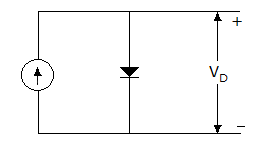Solution:

Id = Io(eVD/ηVT - 1)

By considering, then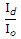= eVD/ηVT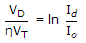Id is constant according to question,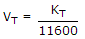VD a T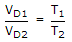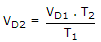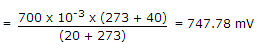.

QUESTION: 25

The work function of a photo surface whose threshold wave length is 1200 A, will be

Solution: